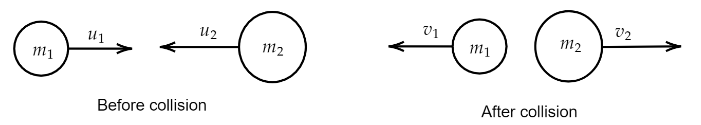Courses
Courses for Kids
Free study material
Free LIVE classes
MoreLIVE
Join Vedantu’s FREE Mastercalss

# Two particles undergo a head on elastic collision. Show that their velocity of separation is equal to their velocity of approach.Verified
255k+ views
Hint:It is known that for an elastic collision total energy and momentum due to collision is always conserved. Use conservation of momentum to find the mass of B. The conservation of momentum states that the momentum of a system in collision is always a constant of motion.

Formula used:
The conservation of momentum is given by,
$mv = K$
where, $K$ is some constant and $mv$ is the momentum of the body
The conservation of kinetic energy is given by,
$\dfrac{1}{2}m{v^2} = K$
where, $m$ is the mass of the particle and $v$ is the velocity and $K$ is some constant of motion.

We know that the momentum is conserved for an elastic collision. Here, two bodies undergo head to head collision. We have to find the relation between the velocity of the bodies before and after the collision. So, let the velocity of the first body before and after collision is ${u_1}$ and ${v_1}$ and let velocity of the second body is ${u_2}$ and ${v_2}$. And the masses are ${m_1},{m_2}$.So, from conservation of momentum we can write, the momentum before collision is equal to momentum after collision. Hence,
${m_1}{u_1} + {m_2}{u_2} = {m_1}{v_1} + {m_2}{v_2}$
$\Rightarrow {m_1}{u_1} - {m_1}{v_1} = {m_2}{v_2} - {m_2}{u_2}$
$\Rightarrow {m_1}({u_1} - {v_1}) = {m_2}({v_2} - {u_2})$….(i)
And, from the conservation of kinetic energy we can write,
$\dfrac{1}{2}{m_1}{u_1}^2 + \dfrac{1}{2}{m_2}{u_2}^2 = \dfrac{1}{2}{m_1}{v_1}^2 + \dfrac{1}{2}{m_2}{v_2}^2$
$\Rightarrow {m_1}({u_1}^2 - {v_1}^2) = {m_2}({v_2}^2 - {u_2}^2)$
$\Rightarrow {m_1}({u_1} + {v_1})({u_1} - {v_1}) = {m_2}({v_2} + {u_2})({v_2} - {u_2})$…..(ii)

So, dividing equation (i) and equation (ii) we will have,
$\dfrac{{{m_1}({u_1} + {v_1})({u_1} - {v_1})}}{{{m_1}({u_1} - {v_1})}} = \dfrac{{{m_2}({v_2} + {u_2})({v_2} - {u_2})}}{{{m_2}({v_2} - {u_2})}}$
$\Rightarrow \dfrac{{({u_1} + {v_1})({u_1} - {v_1})}}{{({u_1} - {v_1})}} = \dfrac{{({v_2} + {u_2})({v_2} - {u_2})}}{{({v_2} - {u_2})}}$
$\Rightarrow ({u_1} + {v_1}) = ({v_2} + {u_2})$
[Since, the initial and final velocities of the particles are not zero so, $({v_2} - {u_2}) \ne 0,({u_1} - {v_1}) \ne 0$]
${u_1} - {u_2} = {v_2} - {v_1}$
Which is the relation between the relative velocity of the particles before and after the collision.Now also we know that the velocity of approach is nothing but the relative velocity of the particles before collision and the velocity of separation is nothing but the relative velocity of the particles after collision.
The relative velocity before collision is,
${u_{rel}} = {u_1} - {u_2}$
The relative velocity after collision is,
${v_{rel}} = {v_1} - {v_2}$
Hence, from equation (iii) we can write,
${u_{rel}} = - {v_{rel}}$
$\therefore \left| {{u_{rel}}} \right| = \left| {{v_{rel}}} \right|$

Hence, it is proved that the velocity of approach is equal to the velocity of separation.

Note: This is only valid when the collision is an elastic collision meaning the forces acting on the particles are only conservative types of forces. If the collision is not elastic then the ratio of the relative velocity will be less than one and this constant is called the coefficient of restitution, whose value depends on the type of the collision.
Last updated date: 22nd Sep 2023
Total views: 255k
Views today: 7.55k